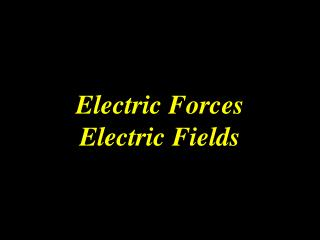DownloadDownload PresentationElectric Forces Electric Fields

# Electric Forces Electric Fields

Download Presentation## Electric Forces Electric Fields

- - - - - - - - - - - - - - - - - - - - - - - - - - - E N D - - - - - - - - - - - - - - - - - - - - - - - - - - -
##### Presentation Transcript

1. Electric ForcesElectric Fields

2. In this section: • 1) Coulomb’s Law

3. Coulomb’s Law

4. Coulomb’s Law Recall that a force is defined as the cause of a change in motion. When two charged objects near one another may experience motion either toward or away from each other, each object exerts a force on the other object. This is called the electric force. force is a vector quantity; has magnitude and direction The fundamental rules of charge interaction applies Opposites attract, like repels like.

5. Coulomb’s Law Charles Coulomb, 1780’s Conducted a variety of experiments to determine the magnitude of the electric force between two charged objects. Coulomb found that the electric force between two charged objects is proportional to the product of the two charges.

6. Coulomb’s Law A torsion balance consists of two small spheres fixed to the ends of a light horizontal rod. The rod is made of an insulating material and is suspended by a silk thread. Coulomb’s torsion balance was used to establish the inverse square law for the electric force between two charges. www.magnet.fsu.edu

7. Coulomb’s Law • Charges are identified as either q+ or q− • Direction of the force depends on whether the charges have the same sign, or opposite signs. ffden-2.phys.uaf.edu

8. Coulomb’s Law • Coulomb discovered • Electric force is proportional to the product of the 2 charges. Hence, if one charge is doubled, the force doubles. If both charges are doubled, the force quadruples. • Electric force is inversely proportional to the square of the distance between the charges. When the distance is halved, the force increases by a factor of 4.

9. Coulomb’s Law where The symbol kC, called the Coulomb constant, and has units of measurement N•m2/C2 because this gives N as the unit of electric force.

10. Coulomb’s Law where This equation satisfies Newton’s 3rd Law because it implies that exactly the same force acts on both charges.

11. Coulomb’s Law Coulombs Law equation describes a force of infinite range which obeys the inverse square law and is of the same form as the Newton’s Law of Universal Gravitation Unit conversions G mC μC nC

12. Coulomb’s Law What is the net force on the third charge? F1,3= (8.99 * 109 N m2/C2) * (75 * 10-3 C * 45 * 10-3 C) / (1.5 m)2 Fon middle due to L = 1.35 * 107 N, positive, pointing to the R Fon middle due to R = (9 * 109 N m2/C2) * (-90 * 10-3 C * 45 * 10-3 C) / (1.5 m)2 Fon middle due to R = -1.62 * 107 N, negative, pointing to the L Net Force = Fon middle due to L + Fon middle due to R Net Force = 1.35 * 107 N - 1.62 * 107 N Net Force = -0.27 * 107 N, pointing left This is the method to solve any Force or E field problem with multiple charges! 1.5 m 1.5 m +75 mC +45 mC −90 mC q1 q2 q3